## Course content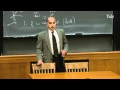1h06m

### 1. Electrostatics

For more information about Professor Shankar's book based on the lectures from this course, Fundamentals of Physics: Mechanics, Relativity, and Thermodynamics, visit http://bit.ly/1jFIqNu.

Fundamentals of Physics, II (PHYS 201)

The course begins with a discussion of electricity. The concept of charge is introduced, and the properties of electrical forces are compared with those of other familiar forces, such as gravitation. Coulomb's Law, along with the principle of superposition, allows for the calculation of electrostatic forces from a given charge distribution.

00:00 - Chapter 1. Review of Forces and Introduction to Electrostatic Force
15:20 - Chapter 2. Coulomb's Law
21:09 - Chapter 3. Conservation and Quantization of Charge
26:15 - Chapter 4. Microscopic Understanding of Electrostatics
33:21 - Chapter 5. Charge Distributions and the Principle of Superposition

Complete course materials are available at the Open Yale Courses website: http://open.yale.edu/courses

This course was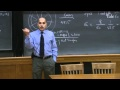1h13m

### 2. Electric Fields

For more information about Professor Shankar's book based on the lectures from this course, Fundamentals of Physics: Mechanics, Relativity, and Thermodynamics, visit http://bit.ly/1jFIqNu.

Fundamentals of Physics, II (PHYS 201)

The electric field is introduced as the mediator of electrostatic interactions: objects generate the field which permeates all of space, and charged objects in the field experience a force with magnitude proportional to their charge. Several instructive examples are given, including the field of an electric dipole and the notion of the electric dipole and dipole moment. The notion of field lines is introduced.

00:00 - Chapter 1. Review of Charges
16:34 - Chapter 2. Electric Fields
33:55 - Chapter 3. Electric Field Lines
40:18 - Chapter 4. Electric Dipoles

Complete course materials are available at the Open Yale Courses website: http://open.yale.edu/courses

This course was recorded in Spring 2010.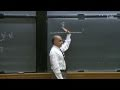1h11m

### 3. Gauss's Law I

For more information about Professor Shankar's book based on the lectures from this course, Fundamentals of Physics: Mechanics, Relativity, and Thermodynamics, visit http://bit.ly/1jFIqNu.

Fundamentals of Physics, II (PHYS 201)

The electric field is discussed in greater detail and field due an infinite line charge is computed. The concepts of charge density and electric flux are introduced and Gauss's Law, which relates the two, is derived. It is applied to the study of the electric field generated by a spherical charge distribution.

00:00 - Chapter 1. Review of Electric field concepts
15:02 - Chapter 2. Electric field due to an infinite line of charge
28:41 - Chapter 3. The Infinite Sheet and Charge Density
44:29 - Chapter 4. Gauss' Law

Complete course materials are available at the Open Yale Courses website: http://open.yale.edu/courses

This course was recorded in Spring 2010.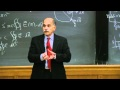1h15m

### 4. Gauss's Law and Application to Conductors and Insulators

For more information about Professor Shankar's book based on the lectures from this course, Fundamentals of Physics: Mechanics, Relativity, and Thermodynamics, visit http://bit.ly/1jFIqNu.

Fundamentals of Physics, II (PHYS 201)

Lecture begins with a recap of Gauss's Law, its derivation, its limitation and its applications in deriving the electric field of several symmetric geometries—like the infinitely long wire. The electrical properties of conductors and insulators are discussed. Multiple integrals are briefly reviewed.

00:00 - Chapter 1. Derivation of Gauss' Law
21:12 - Chapter 2. The Electric Field due to a Spherical Distribution of Charge
44:47 - Chapter 3. Electric Field due to an Infinitely Long Wire
51:39 - Chapter 4. Electric Conductors and Insulators

Complete course materials are available at the Open Yale Courses website: http://open.yale.edu/courses

This course was recorded in Spring 2010.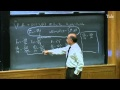1h14m

### 5. The Electric Potential and Conservation of Energy

For more information about Professor Shankar's book based on the lectures from this course, Fundamentals of Physics: Mechanics, Relativity, and Thermodynamics, visit http://bit.ly/1jFIqNu.

Fundamentals of Physics, II (PHYS 201)

The law of conservation of energy is reviewed using examples drawn from Newtonian mechanics. The work-energy theorem is derived from first principles and used to initiate a discussion of the vector calculus underlying the law of conservation of energy.

00:00 - Chapter 1. Review of Electrostatics
03:49 - Chapter 2. Review of Law of Conservation of Energy
08:13 - Chapter 3. Deriving the Work-Energy Theorem and the Law of Conservation of Energy
59:33 - Chapter 4. Electric Potential

Complete course materials are available at the Open Yale Courses website: http://open.yale.edu/courses

This course was recorded in Spring 2010.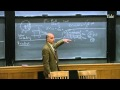1h12m

### 6. Capacitors

For more information about Professor Shankar's book based on the lectures from this course, Fundamentals of Physics: Mechanics, Relativity, and Thermodynamics, visit http://bit.ly/1jFIqNu.

Fundamentals of Physics, II (PHYS 201)

The electric potential is defined for the electric field. It is introduced as an integral of the electric field making the field the derivative of the potential. After discussing the ideas of electric potential and field as presented in the previous lecture, the concept of capacitance is introduced as a means of storing charge and energy.

00:00 - Chapter 1. Review of Electric Potential
15:52 - Chapter 2. Advantages of Electric Potential, V
43:31 - Chapter 3. Conductors as Equipotentials
61:46 - Chapter 4. Capacitors

Complete course materials are available at the Open Yale Courses website: http://open.yale.edu/courses

This course was recorded in Spring 2010.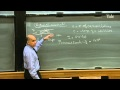1h14m

### 7. Resistance

For more information about Professor Shankar's book based on the lectures from this course, Fundamentals of Physics: Mechanics, Relativity, and Thermodynamics, visit http://bit.ly/1jFIqNu.

Fundamentals of Physics, II (PHYS 201)

Lecture begins with a discussion of electric potential distribution in conductors. Image charges are introduced and exploited. Capacitance is explained in greater detail and illustrated using the parallel plate capacitor. The energy stored in the electric field is derived. The forces acting on an electric current flowing through a conducting wire are examined. The RC circuits and its energetics are discussed. The EMF due to a battery is explained.

00:00 - Chapter 1. Review of Image Charges
28:45 - Chapter 2. The Parellel Plate Capacitor
42:46 - Chapter 3. The Concept of Resistance

Complete course materials are available at the Open Yale Courses website: http://open.yale.edu/courses

This course was recorded in Spring 2010.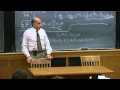1h12m

### 8. Circuits and Magnetism I

For more information about Professor Shankar's book based on the lectures from this course, Fundamentals of Physics: Mechanics, Relativity, and Thermodynamics, visit http://bit.ly/1jFIqNu.

Fundamentals of Physics, II (PHYS 201)

After a description of more complicated electric circuits, the basic ideas underlying magnetism are discussed and the relationship between electrical charges and magnetic fields is explored. Magnetism is caused and experienced only by moving charges. The Lorentz force on a charge is described and used to deduce the force on a current carrying wire. The cyclotron and velocity selector are described.

00:00 - Chapter 1. Review of Electric Circuits
34:32 - Chapter 2. Introduction to Magnetism
45:29 - Chapter 3. Fundamental Equations of Magnetostatics
62:42 - Chapter 4. Force on a Current Carrying Wire

Complete course materials are available at the Open Yale Courses website: http://open.yale.edu/courses

This course was recorded in Spring 2010.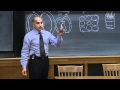1h11m

### 9. Magnetism II

For more information about Professor Shankar's book based on the lectures from this course, Fundamentals of Physics: Mechanics, Relativity, and Thermodynamics, visit http://bit.ly/1jFIqNu.

Fundamentals of Physics, II (PHYS 201)

The mechanism by which electric currents produce a magnetic field (Law of Biot-Savart) is discussed in greater detail. The field due to a single loop and an infinite wire are computed. Ampere's Law is derived. The operation of the DC electric motor is used to illustrate the torque generated on moving charges in a magnetic field.

00:00 - Chapter 1. Review of Magnetic Fields
14:00 - Chapter 2. Torque on Charge moving in Magnetic Field
20:56 - Chapter 3. Magnetic effects produced by electric currents
51:26 - Chapter 4. Ampere's Law

Complete course materials are available at the Open Yale Courses website: http://open.yale.edu/courses

This course was recorded in Spring 2010.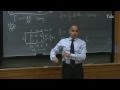1h10m

### 10. Ampere's Law

For more information about Professor Shankar's book based on the lectures from this course, Fundamentals of Physics: Mechanics, Relativity, and Thermodynamics, visit http://bit.ly/1jFIqNu.

Fundamentals of Physics, II (PHYS 201)

Ampere's Law is used to find the magnetic field generated by currents in highly symmetric geometries like the infinitely long wire and the solenoid. It is shown how magnetism can be used to convert macroscopic mechanical energy to do microscopic electrical work. Lenz's and Faraday's Laws are introduced. The latter says that a changing magnetic field generates a non-conservative electric field.

00:00 - Chapter 1. Review of Ampere's Law
08:46 - Chapter 2. Magnetic field generated by current in a solenoid
49:51 - Chapter 3. Lenz's Law
67:07 - Chapter 4. Faraday's Law

Complete course materials are available at the Open Yale Courses website: http://open.yale.edu/courses

This course was recorded in Spring 2010.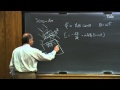1h09m

### 11. Lenz's and Faraday's Laws

For more information about Professor Shankar's book based on the lectures from this course, Fundamentals of Physics: Mechanics, Relativity, and Thermodynamics, visit http://bit.ly/1jFIqNu.

Fundamentals of Physics, II (PHYS 201)

The electric effect of a changing magnetic field is described using Faraday's Law. The direction of the current so generated is given by Lenz's Law. The operation and energy accounting of the generator are described. The concept of inductance is introduced. The Betatron is described as an example of Faraday's Law. Self and mutual inductance are introduced. The energy density in a magnetic field is derived.

00:00 - Chapter 1. Review of Lenz and Faraday's Law
25:37 - Chapter 2. The power generator
37:45 - Chapter 3. Mutual and self inductance
64:10 - Chapter 4. Energy density of a magnetic field

Complete course materials are available at the Open Yale Courses website: http://open.yale.edu/courses

This course was recorded in Spring 2010.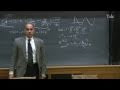1h09m

### 12. LCR Circuits—DC Voltage

For more information about Professor Shankar's book based on the lectures from this course, Fundamentals of Physics: Mechanics, Relativity, and Thermodynamics, visit http://bit.ly/1jFIqNu.

Fundamentals of Physics, II (PHYS 201)

Like capacitors, inductors act as energy storage devices in circuits. The relationship between voltage, inductance and current in a variety of circuits with DC voltages is described.

00:00 - Chapter 1. Review of Inductors
04:46 - Chapter 2. Inductive Circuits
54:18 - Chapter 3. LCR Circuits driven by an Alternating Source

Complete course materials are available at the Open Yale Courses website: http://open.yale.edu/courses

This course was recorded in Spring 2010.

For more information about Professor Shankar's book based on the lectures from this course, Fundamentals of Physics: Mechanics, Relativity, and Thermodynamics, visit http://bit.ly/1jFIqNu.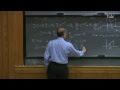1h10m

### 13. LCR Circuits—AC Voltage

For more information about Professor Shankar's book based on the lectures from this course, Fundamentals of Physics: Mechanics, Relativity, and Thermodynamics, visit http://bit.ly/1jFIqNu.

Fundamentals of Physics, II (PHYS 201)

The mathematics underlying LCR circuit theory for AC currents is discussed. Complex numbers are used to convert differential equations to algebraic equations. The notion of impedance is introduced. The radio is used to illustrate the concepts of resonance and variable capacitance. The body of classical electromagnetism treated so far is reviewed and summarized. The displacement current is introduced, leading to the complete Maxwell equations.

00:00 - Chapter 1. Review of LCR Circuits
08:48 - Chapter 2. Impedance
17:39 - Chapter 3. Resonance and Variable Capacitance
68:03 - Chapter 4. Displacement current

Complete course materials are available at the Open Yale Courses website: http://open.yale.edu/courses

This course was recorded in Spring 2010.

For more i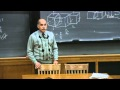1h09m

### 14. Maxwell's Equations and Electromagnetic Waves I

For more information about Professor Shankar's book based on the lectures from this course, Fundamentals of Physics: Mechanics, Relativity, and Thermodynamics, visit http://bit.ly/1jFIqNu.

Fundamentals of Physics, II (PHYS 201)

Waves on a string are reviewed and the general solution to the wave equation is described. Maxwell's equations in their final form are written down and then considered in free space, away from charges and currents. It is shown how to verify that a given set of fields obeys Maxwell's equations by considering them on infinitesimal cubes and loops. A simple form of the solutions is assumed and the parameters therein fitted using Maxwell's equations. The wave equation follows, along with the wave speed equal to that of light (3 x 10^8), suggesting (correctly) that light is an electromagnetic wave. The vector relationship between the electric field, the magnetic field and the direction of wave propagation is described.

00:00 - Chapter 1. Background
04:43 - Chapter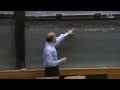1h09m

### 15. Maxwell's Equations and Electromagnetic Waves II

For more information about Professor Shankar's book based on the lectures from this course, Fundamentals of Physics: Mechanics, Relativity, and Thermodynamics, visit http://bit.ly/1jFIqNu.

Fundamentals of Physics, II (PHYS 201)

The physical meaning of the components of the wave equation and their applications are discussed. The power carried by the wave is derived. The fact that, unlike Newton's laws, Maxwell's equations are already consistent with relativity is discussed. The existence of magnetism is deduced from a thought experiment using relativity.

00:00 - Chapter 1. Recap—Solving Maxwell's Equations
18:18 - Chapter 2. Deriving the Energy and Intensity of an Electromagnetic Wave
30:40 - Chapter 3. The Origin of Electromagnetic Waves
37:03 - Chapter 4. Relativity and Maxwell's Equations
51:44 - Chapter 5. Deducing the Presence of Magnetism

Complete course materials are available at the Open Yale Courses website: http://open.yale.edu/courses

This course was recorded in Spring 2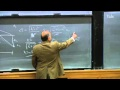1h13m

### 16. Ray or Geometrical Optics I

For more information about Professor Shankar's book based on the lectures from this course, Fundamentals of Physics: Mechanics, Relativity, and Thermodynamics, visit http://bit.ly/1jFIqNu.

Fundamentals of Physics, II (PHYS 201)

Geometric optics is discussed as an approximation to wave theory when the wavelength is very small compared to other lengths in the problem (such as the size of openings). Many results of geometric optics involving reflection, refraction (mirrors and lenses) are derived in a unified way using Fermat's Principle of Least Time.

00:00 - Chapter 1. Light as an Electromagnetic Phenomenon
07:17 - Chapter 2. Review of Geometrical (Classical) Optics
21:50 - Chapter 3. Fermat's Principle of Least Time and its Corollaries

Complete course materials are available at the Open Yale Courses website: http://open.yale.edu/courses

This course was recorded in Spring 2010.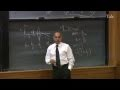1h11m

### 17. Ray or Geometrical Optics II

For more information about Professor Shankar's book based on the lectures from this course, Fundamentals of Physics: Mechanics, Relativity, and Thermodynamics, visit http://bit.ly/1jFIqNu.

Fundamentals of Physics, II (PHYS 201)

Ray diagrams are used to investigate the behavior of light incident on mirrors and lenses. The principle of least time is used to show that all rays from an object in front of a concave mirror focus on the image point if they are not too far from the axis. The experiments describing the breakdown of geometric optics are discussed.

00:00 - Chapter 1. Parabolic and Spherical Mirrors
34:16 - Chapter 2. Lenses
43:34 - Chapter 3. Focal Point
63:56 - Chapter 4. Magnifying Lenses

Complete course materials are available at the Open Yale Courses website: http://open.yale.edu/courses

This course was recorded in Spring 2010.

For more information about Professor Shankar's book based on the lectures from this course, Fundamentals of Physics: Mechanics, Relativity, and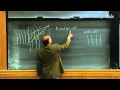1h14m

### 18. Wave Theory of Light

For more information about Professor Shankar's book based on the lectures from this course, Fundamentals of Physics: Mechanics, Relativity, and Thermodynamics, visit http://bit.ly/1jFIqNu.

Fundamentals of Physics, II (PHYS 201)

Young's double slit experiment shows clearly that light is a wave. (In order to observe the wave behavior of light, the slit size and separation should be comparable or smaller than the wavelength of light.) Interference is described using real and complex numbers (in anticipation of quantum mechanics). Grating and crystal diffraction are analyzed.

00:00 - Chapter 1. Revisions to Geometric Optics
08:20 - Chapter 2. Young's double slit experiment
50:52 - Chapter 3. Interference and Diffraction of Light

Complete course materials are available at the Open Yale Courses website: http://open.yale.edu/courses

This course was recorded in Spring 2010.

For more information about Professor Shankar's book based on the lectures from this course, Fundamentals of Physic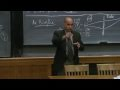1h13m

### 19. Quantum Mechanics I: The key experiments and wave-particle duality

For more information about Professor Shankar's book based on the lectures from this course, Fundamentals of Physics: Mechanics, Relativity, and Thermodynamics, visit http://bit.ly/1jFIqNu.

Fundamentals of Physics, II (PHYS 201)

The double slit experiment, which implies the end of Newtonian Mechanics is described. The de Broglie relation between wavelength and momentum is deduced from experiment for photons and electrons. The photoelectric effect and Compton scattering, which provided experimental support for Einstein's photon theory of light are reviewed. The wave function is introduced along with the probability interpretation. The uncertainty principle is shown arise from the fact that the particle's location is determined by a wave and that waves diffract when passing a narrow opening.

00:00 - Chapter 1. Recap of Young's double slit experiment
09:10 - Chapter 2. The Particulate Nature of Light
23:15 - Chapter 3. The Photoelectric Effect
31:19 - Chapter 4. Compton's scattering
36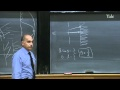1h15m

### 20. Quantum Mechanics II

For more information about Professor Shankar's book based on the lectures from this course, Fundamentals of Physics: Mechanics, Relativity, and Thermodynamics, visit http://bit.ly/1jFIqNu.

Fundamentals of Physics, II (PHYS 201)

Lecture begins with a detailed review of the double slit experiment with electrons. The fate of an electron traversing the double slit is determined by a wave putting an end to Newtonian mechanics. The momentum and position of an electron cannot both be totally known simultaneously. The wave function is used to describe a probability density function for an electron. Heuristic arguments are given for the wave function describing a particle of definite momentum.

00:00 - Chapter 1. Review of Double Slit Experiment using Electrons
20:28 - Chapter 2. Heisenberg's Uncertainty Principle
42:32 - Chapter 3. The Probability Density Function of an Electron

Complete course materials are available at the Open Yale Courses website: http://open.yale.edu/courses

This cou